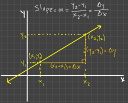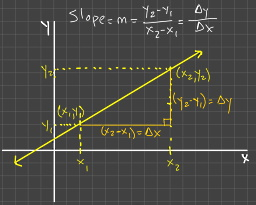﻿ What is Slope? What does it tell you?
sin^2x + cos^2x = 1
y = frac (-b +- sqrt(b^2 - 4ac)) (2a)
(x + 2)^2 + (y - 3)^2 = 16
slope = m = frac (\text(change in y)) (\text(change in x)) = frac (Deltay) (Deltax)

# Get Math Help!### REGISTER ON GMH

* = required field
<< Back to Blog

### Everything's Relative (frac (Deltay) (Deltax))

#### What is Slope? What does it tell you?

Ã—###### What is slope?

Slope is one of the most versatile and applicable concepts in mathematics. In its simplest form, it's just two numbers and a division operation, a fraction, and yet it's the basis for all of Calculus!

###### What does it tell you?

If you have two variables that are related such that the value of the one is dependent on the value of the other, then the slope of their relationship tells you how much the value of the dependent variable (typically y) will change if the value of the independent variable (typically x) changes. It defines a measure of the relationship between these two variables. In mathematics it is standard practice to use the letter m to represent slope so when you see m, think slope.

###### How is it calculated?

The slope of a linear functionA linear function has degree 1, and its graph is a line., y=f(x), is found by evaluating the fraction (text(change in y))/(text(change in x)). If we know two points, say (x_1,y_1) and (x_2,y_2), that lie on the graph of the functionIf a point lies on the graph of a function, then the point's x and y coordinates satisfy the equation y=f(x)., f(x), then we can calculate the slope of that line by finding the text((change in y))  = (y_2 - y_1) and the text((change in x)) = (x_2 - x_1) and then evaluating the fraction, (y_2 - y_1)/(x_2 - x_1) = mAgain, m stands for slope..

###### What I'd like for you to forget and Why slope is so important!

Many high school math teachers like to tell their students that a good way to remember slope is to remember text(rise-over-run). It's got some cool alliteration, and it's short and sweet. They think it has a lot going for it; what's not to like? I know; rise is the movement along the vertical axis, so it's change-in-y and run is the movement along the horizontal axis, so it's change-in-x. It seems like it's the same thing I'm saying, but what if you have a line with a negative slope? Then either the rise is really a fall, or you're running backwards. It just doesn't work as well with a negative slope; the coolness is gone.

The main reason I'd like for you to forget text(rise-over-run) is the role slope plays in more advanced mathematics, particularly Calculus. Calculus is the mathematics of change. In my opinion, slope is the most important concept to understand to do well in calculus. Calculus is based on the concept of slope. When I teach my Calculus I course at Harford Community College, I probably say the word, 'slope', at least 10 times in every class. Without slope, you can't have calculus. Suppose you have a cost function, C(x) = 3x^3 - 5x^2 + 7x - 20, for some product that is being manufactured, and you're asked to minimize the production cost of that product, text(rise-over-run) seems unrelated to this problem unless you graph the cost function first. There is no need to graph it; it's just unnecessary, extra work. But if you understand functions, you know that C(x) is another name for y. Calculus is all about determining how the value of one variable changes when a change in the value of another variable occurs. So thinking (text(change in y))/(text(change in x)) makes perfect sense and is exactly what you need. You need to work with (text(change in y))/(text(change in x)) = (text(change in ) C(x))/text(change in x).

Differential Calculus starts with the slope of a line and then extends that to the slope of a curve by introducing the concept of limits. That is used to define the derivative which gives you the slope of a curve at any point on the curve.

lim_(Deltax rightarrow 0) (Deltay)/(Deltax) = lim_(Deltax rightarrow 0) ( (text(change in ) y)/(text(change in ) x) )The Greek letter Delta is used as an abbreviation for 'change in' in mathematics. So Deltax means 'change in x'.

You can't get there if you think slope is text(rise-over-run).#### Popular Tags

here are the popular tags...